# RD Sharma Class 9 Solutions Chapter 3 Rationalisation Ex 3.1

## RD Sharma Class 9 Solutions Chapter 3 Rationalisation Ex 3.1

These Solutions are part of RD Sharma Class 9 Solutions. Here we have given RD Sharma Class 9 Solutions Chapter 3 Rationalisation Ex 3.1

Question 1.
Simplify each of the following: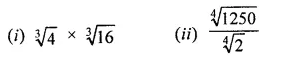Solution: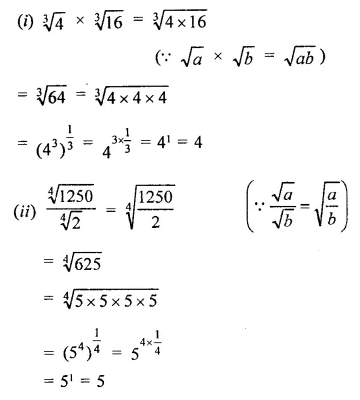Question 2.
Simplify the following expressions:
(i)  (4 + $$\sqrt { 7 }$$) (3 + $$\sqrt { 2 }$$)
(ii) (3 + $$\sqrt { 3 }$$ )(5- $$\sqrt { 2 }$$)
(iii) ($$\sqrt { 5 }$$ -2)($$\sqrt { 3 }$$ – $$\sqrt { 5 }$$)
Solution: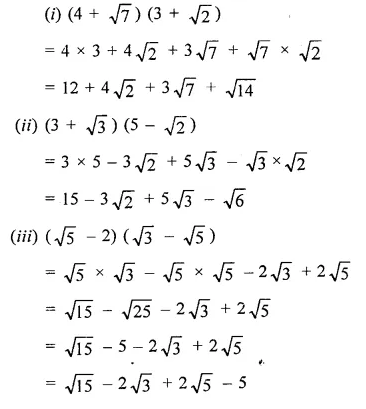Question 3.
Simplify the following expressions:
(i)  (11 + $$\sqrt { 11 }$$) (11 – $$\sqrt { 11 }$$)
(ii) (5 + $$\sqrt { 7 }$$ ) (5 – $$\sqrt { 7 }$$ )
(iii) ($$\sqrt { 8 }$$ – $$\sqrt { 2 }$$) ($$\sqrt { 8 }$$+ $$\sqrt { 2 }$$)
Solution: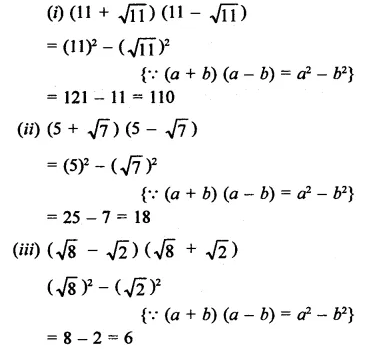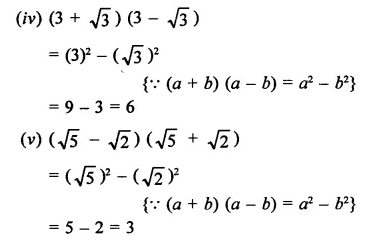Question 4.
Simplify the following expressions:
(i) ($$\sqrt { 3 }$$+$$\sqrt { 7 }$$)2
(ii) ($$\sqrt { 5 }$$ – $$\sqrt { 3 }$$ )2
(iii) (2$$\sqrt { 5 }$$ + 3 $$\sqrt { 2}$$)2
Solution:
(i)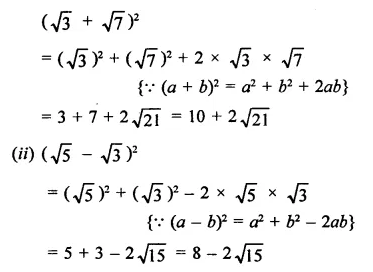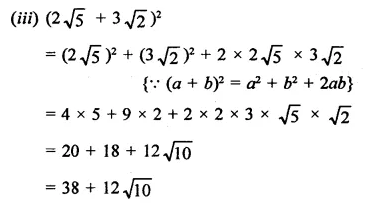Hope given RD Sharma Class 9 Solutions Chapter 3 Rationalisation Ex 3.1 are helpful to complete your math homework.

If you have any doubts, please comment below. Learn Insta try to provide online math tutoring for you.